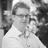# Alternating current and its relation to voltage

Published on 05 January 2023, updated on 10 January 2023 -

## Intro

For a while now I am interested in electronics. So after some false starts, I started following a long course on it: Crash Course Electronics and PCB Design.

Lecture 9 was about alternating current (AC), the kind of current that flows from a wall socket. I struggled to understand how it's voltage is determined, so I took a lot of notes. This is the result.

Warning: algebra ahead. Also: everything here may be wildly inaccurate. Did I mention that I am just learning this stuff?

## What is alternating current

Alternating current measn that the supply source voltage changes as a function of time. In general, this is a sine wave, with positive high peak voltages and negative low peak voltages.

A negative voltage means that the current flows in the opposite direction, from minus (-) to plus (+). In the span of a full sine wave, the current changes direction twice. So with a standard 60Hz alternating current, the current changes direction 120 times per second.

## Properties of alternating current

Alternating current has a few commonly-used properties:

• Peak voltage: the peak voltages on both the upper peak ($V_{pmax}$) and lower peak ($V_{pmin}$).
• Peak to peak voltage: the voltage difference between the two peaks: $V_{pp} = V_{pmax} - V_{pmin}$.
• Frequency: the number of complete cycles (of $V_{pmax}$ and $V_{pmin}$) per second.
• Root-mean-square voltage: the voltage calculation usually associated with alternating current, e.g. 230V or 120V.

In the rest of this note, we will work with an AC voltage with the following properties:

## Root Mean Square Voltage

Root Mean Square (RMS, wikipedia) is a formula that is often used whencalculating the voltage over time for an alternating current circuit.

Its definition is as follows:

$$f(rms) = \lim_{T \to \infty} \sqrt{\frac{1}{T} \int_0^T [f(t)]^2 dt}$$

For a pure sine wave, which is common, the formula for the peak-to-peak amplitude can be simplified as:

$$V_{pp} = 2\sqrt{2} \cdot RMS \approx 2.828 \cdot RMS$$

### Alternate ways of writing

That also means that

• $V_{rms} = V_{pp} \div 2\sqrt{2} = \frac{V_{pp}}{2\sqrt{2}}$
• Written differently: $V_{rms} = V_{pp} \cdot \frac{1}{2\sqrt{2}} = V_{pp} \cdot \frac{1}{2} \cdot \frac{1}{\sqrt{2}}$
• This works, because we can pull out the $\frac{1}{2}$ out of $\frac{1}{2\sqrt{2}}$, as long as we then multiply by the remainder ($\frac{1}{\sqrt{2}}$), which, again, then gives in full: $V_{rms} = V_{pp} \cdot \frac{1}{2} \cdot \frac{1}{\sqrt{2}}$.

I know, this is high school level algebra, but it's been a long time and I struggled to get there. So I wrote it out in full.

So, when calculating, one then gets:

• $V_{rms} = V_{pp} \cdot \frac{1}{2} \cdot \frac{1}{\sqrt{2}}$
• $V_{rms} \approx V_{pp} \cdot 0.5 \cdot \frac{1}{1.414}$
• $V_{rms} \approx V_{pp} \cdot 0.5 \cdot 0.707$
• $V_{rms} \approx V_{pp} \cdot 0.354$

### Example calculations

With wo common voltages 120V RMS and 230V RMS, we get:

• For 120V RMS: $V_{pp} \approx 2.828 \cdot 120 \approx 339V$
• For 230V RMS: $V_{pp} \approx 2.828 \cdot 230 \approx 650V$

And when we go back:

• For 120V RMS: $V_{rms} \approx V_{pp} \cdot .354 \approx 339 \cdot .354 \approx 120V$
• For 230V RMS: $V_{rms} \approx V_{pp} \cdot .354 \approx 650 \cdot .354 \approx 230V$

Given a symmetrical sine wave with the reference point as 0V, that gives also:

• For 120V RMS: $V_{pp} = V_{phigh} - V_{plow} \approx 169V - (-169V)$
• For 230V RMS: $V_{pp} = V_{phigh} - V_{plow} \approx 325V - (-325V)$

## Fin

That was it for today. If you found this useful, I highly recommend following the course.